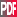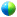18th Conference on Probability and Statistics in the Atmospheric Sciences

4.7

An ensemble data assimilation scheme that has mixed Gaussian and non-Gaussian errors

Steven J. Fletcher, Colorado State University, Fort Collins, CO; and M. Zupanski

Currently under development at the Cooperative Institute for Research in the Atmosphere, CIRA, Colorado State University, CSU, and Florida State University, FSU, is an ensemble filter similar to the Ensemble Transform Kalman Filter, ETKF, where instead of using the ensemble mean to generate the statistics we use the state which minimizes a non-linear quadratic cost function similar to that used in 3D VAR. This state can be considered as the most likely state and hence the new filter is called the Maximum Likelihood Ensemble Filter, MLEF. One of the assumptions made in the Bayesian probability derivation for the cost function is that the background and observational errors are Normally distributed. We have altered this assumption and derived a new cost function assuming that the errors are lognormally distributed. As an extension to this we have been able to combine different errors types mainly Normal and lognormal errors into a new statistical distribution that has the properties of both distribution but allows for outliers that may be assigned a very small probability in a Normal framework. With this new distribution these outliers are assigned a higher probability as the distribution inherits the tail that is associated with the lognormal distribution. Here we present the new distribution and show it properties in a bi-variate case as well as show results from the MLEF with the new cost function associated with this new distribution in CSU's 2D shallow water equations model on the sphere where the initial conditions are different Rossby-Haurwitz waves which generate flows similar to that in the atmosphere in the shallow water equations model.Extended Abstract (124K)Recorded presentation

Session 4, Ensemble Forecasting
Tuesday, 31 January 2006, 8:45 AM-11:45 AM, A304

Previous paper

Browse or search entire meeting